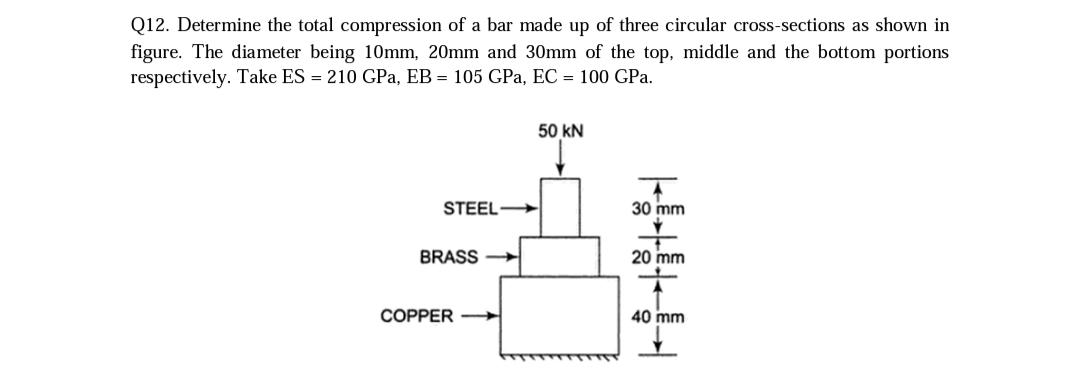# Determine the total compression of a bar made up of three circular cross-sections as shown in figure. The diameter being 10mm, 20mm and 30mm of the top, middle and the bottom portions respectively. Take ES = 210 GPa, EB = 105 GPa, EC = 100 GPa.

Question-AnswerCategory: Strength of MaterialsDetermine the total compression of a bar made up of three circular cross-sections as shown in figure. The diameter being 10mm, 20mm and 30mm of the top, middle and the bottom portions respectively. Take ES = 210 GPa, EB = 105 GPa, EC = 100 GPa.Determine the total compression of a bar made up of three circular cross-sections as shown in figure. The diameter being 10mm, 20mm and 30mm of the top, middle and the bottom portions respectively. Take ES = 210 GPa, EB = 105 GPa, EC = 100 GPa.